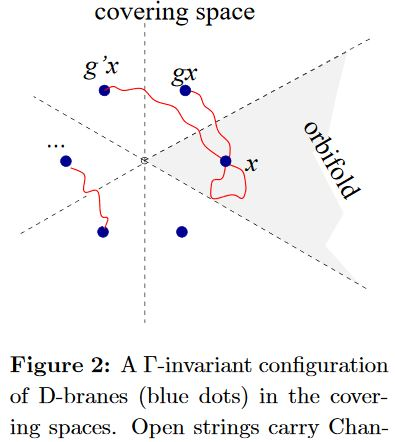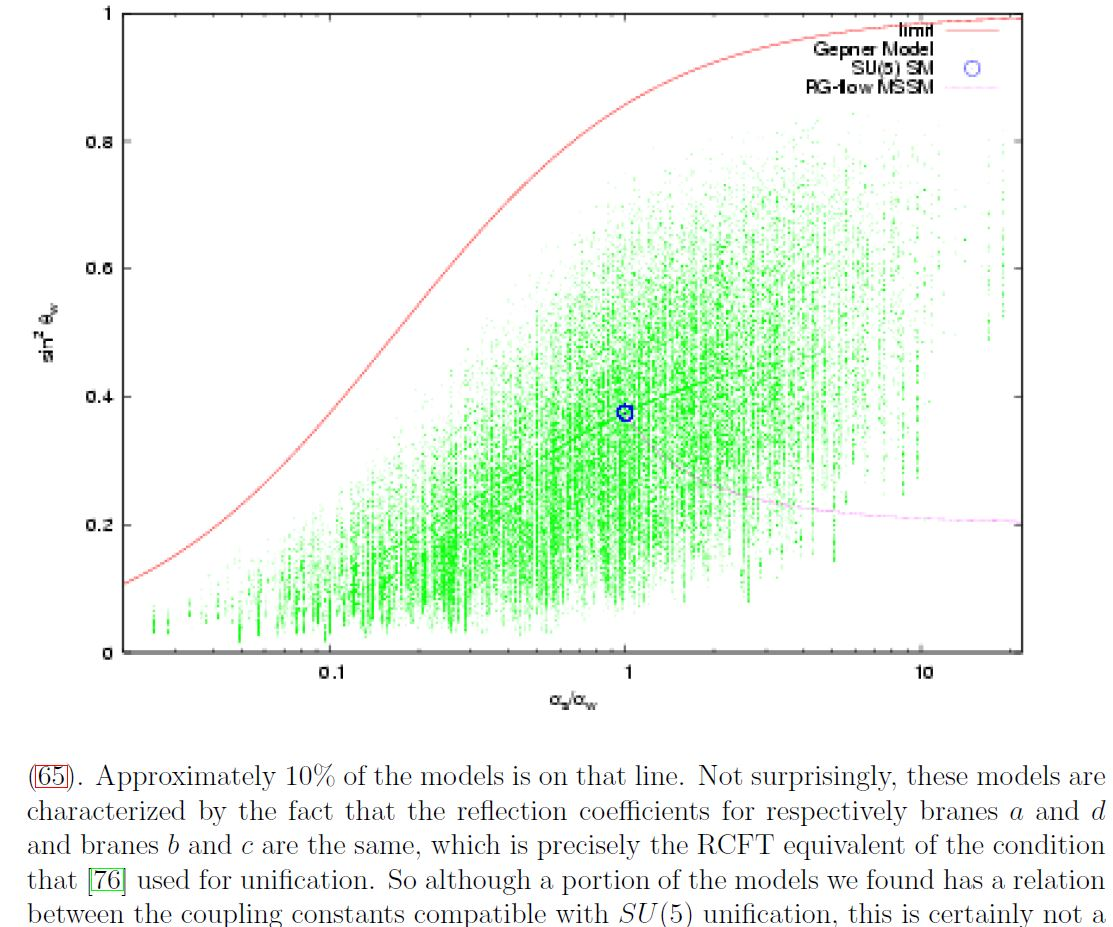Contents

# Contents

## Idea

The study of conformal field theory on manifolds with boundary, hence conformal boundary field theory, is known as boundary conformal field theory (often abbreviated as BCFT).

For the special case of 2d CFT understood as the quantum field theory on the worldsheet of strings, boundary conformal field theory is the key ingredient of perturbative string theory with prescribed boundary conditions for the open strings. From the target spacetime-perspective these boundary conditions are interpreted as the D-branes that the open strings may “end on”.

In this way 2d BCFT can serve to give an algebraic definition of D-branes, independent of or complementary to their incarnation in the target-spacetime geometry. In particular fractional D-branes may be described this way as boundary conditions for the 2d CFTs describing open strings propagating in orbifolds.

A key tool of BCFT is the boundary state formalism, where one interprets boundary conditions for the open string as coherent states of the corresponding closed string 2d CFT. The physical interpretation of this algebraic fact is that the D-brane, being massive (see also at black brane) emits and absorbs gravitons, which, in string theory, are excitations of the closed string.

It has been argued that, therefore, for sigma-model 2d CFTs the classification of equivalence classes of BCFT boundary states under renormalization group flow should reproduce the expected classification of D-branes by the (equivariant, twisted K-theory) topological K-theory of the target spacetime (Moore 03, section 3).

While this gives, in principle, a precise algebraic definition of D-brane charge from the worldsheet-perspective, in practice it is hard to follow the renormalization group flow of the boundary states. The proposal has been checked in some special cases (e.g. Braun & Schaefer-Nameki 05). Other authors reported some discrepancies (Quiroz-Stefanski 01)

Specifically for fractional D-branes at $G$-orbifold singularities the relevant boundary states are sums of boundary states for each “$g$-twisted sector” of the closed string 2d CFT, consisting of those string configurations that are closed in the covering space up to the action of the element $g \in G$:graphics grabbed from BCR 00

Here the coefficient of the $g$-twisted sector in the total boundary state is proprotional to the value $\chi_V(g)$ of the character $\chi_V$ of some $G$-representation $V \in R_{\mathbb{C}}(G) = KU_G(\ast)$ (e.g. Recknagel-Schomerus 13 (4.102)). This makes it plausible that the RG-flow-equivalence classes of the boundary states for fractional D-branes do coincide with the equivariant K-theory $KU_G(\ast) = R_{\mathbb{C}}(G)$ of the orbifold singularity (the representation ring of $G$).

But maybe this has not yet been actually proven?

## Results

There are some mistakes in the literature. A clean account is in Fuchs-Schweigert-Walcher 00, Fuchs-Kaste-Lerche-Lutken-Schweigert 00

## Computer scan of Gepner-model compactifications

Discussion of string phenomenology of intersecting D-brane models KK-compactified with non-geometric fibers such that the would-be string sigma-models with these target spaces are in fact Gepner models (in the sense of Spectral Standard Model and String Compactifications) is in (Dijkstra-Huiszoon-Schellekens 04a, Dijkstra-Huiszoon-Schellekens 04b):A plot of standard model-like coupling constants in a computer scan of Gepner model-KK-compactification of intersecting D-brane models according to Dijkstra-Huiszoon-Schellekens 04b.

The blue dot indicates the couplings in $SU(5)$-GUT theory. The faint lines are NOT drawn by hand, but reflect increased density of Gepner models as seen by the computer scan.

### General

The boundary state formalism in BCFT is due to

• C. G. Callan, C. Lovelace, C. R. Nappi and S. A. Yost, Adding holes and crosscaps to the superstring, Nucl. Phys. B 293 (1987) 83 (doi:10.1016/0550-3213(87)90065-4)

• C. G. Callan, C. Lovelace, C. R. Nappi and S. A. Yost, Loop corrections to superstring equations of motion, Nucl. Phys. B308 (1988) 221 (pdf)

• N. Ishibashi, Boundary and Crosscap States in Conformal Field Theories, Mod. Phys. Lett. A4(1989) 251 (pdf)

• N. Ishibashi and T. Onogi, Open string model building, Nucl. Phys. B318(1989) 239 (doi:10.1016/0550-3213(89)90054-0)

• Dixon-Friedan-Martinec-Shenker 87

A textbook account is in

### Relation to K-theory

The suggestion that renormalization group flow-equivalence classes of boundary states in 2d BCFT should realize the expected classification of D-brane charge in (equivariant) topological K-theory apparntly goes back to

This has been checked in examples in

A mismatch was claimed in

### For rational CFT, WZW models and Gepner models

For rational CFT, specifically Gepner models and WZW models

### On orbifolds

Discussion specifically concerning fractional D-branes on orbifolds includes